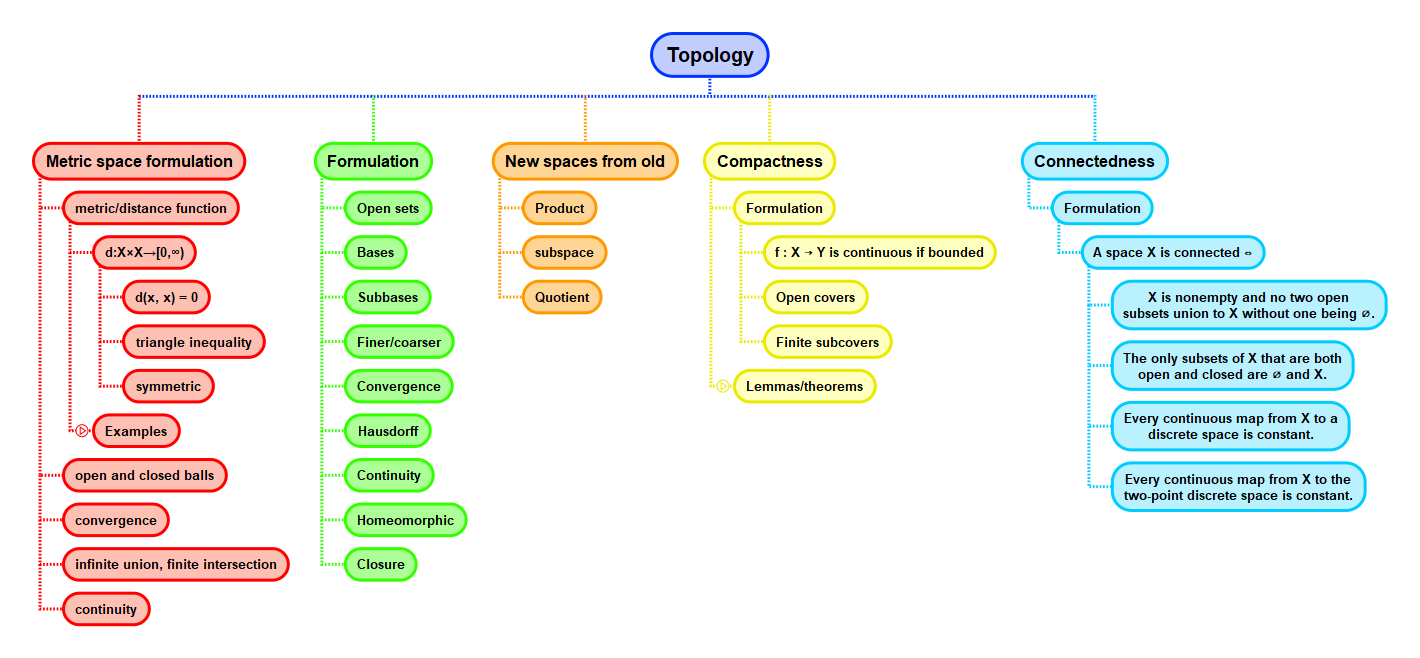Show Question
Math and science::Topology

# Connectedness. Equivalent formulations

For a nonempty topological space $$X$$, the following are equivalent:

1. $$X$$ is connected;
2. the only subsets that are both open and closed are $$\emptyset$$ and $$X$$;
3. every continuous map from $$X$$ to a discrete space is constant;
4. every continuous map from $$X$$ to the two-point discrete space is constant.

The 3rd and 4th statement are similar, yet both useful in their own right: the 3rd statement is general and allows progress to be made in a proof when one knows that $$X$$ is connected. The 4th is powerful in the reverse direction: simply proving that all continuous maps to the two-point discrete space is constant is enough to show that $$X$$ is connected.

## ContextLeinster, p 69